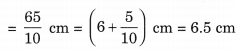# NCERT Solutions for Class 6 Maths Chapter 8 Decimals Ex 8.1

ncert textbook

## NCERT Solutions for Class 6 Maths Chapter 8 Decimals Ex 8.1

NCERT Solutions for Class 6 Maths Chapter 8 Decimals Ex 8.1

Exercise 8.1

Question 1.
Write the following as numbers in the given table.Solution:
From the given data, we have

 Hundreds (100) Tens (10) Ones (1) Tenths (1/10) 0 3 1 2 1 1 0 4

Question 2.
Write the following decimals in the place value table.
(a) 19.4
(b) 0.3
(c) 10.6
(d) 205
Solution:
Place value table is given as under:Question 3.
Write each of the following as decimals:
(a) Seven-tenths
(b) Two tens and nine-tenths
(c) Fourteen point six
(d) One hundred and two ones
(e) Six hundred point eight
Solution:
(a) Seven-tenths = 7 x$\frac { 1 }{ 10 }$= 0.7
(b) Two tens and nine-tenths = 2 tens + 0 ones + 9 tenths = 20 +$\frac { 9 }{ 10 }$= 20.9
(c) Fourteen point six = 14.6
(d) One hundred and two ones
= 1 x 100 + 2 x 1 = 100 + 2 = 102
(e) Six hundred point eight = 600.8

Question 4.
Write each of the following as decimals: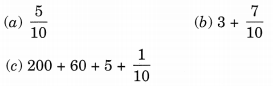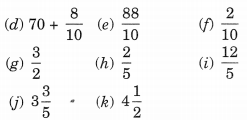Solution:Question 5.
Write the following decimals as fractions. Reduce the fractions to lowest form.
(a) 0.6
(b) 2.5
(c) 1.0
(d) 3.8
(e) 13.7
(f) 21.2
(g) 6.4
Solution: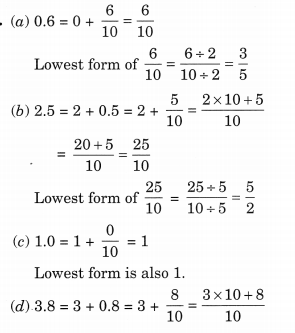Question 6.
Express the following as cm using decimals.
(a) 2 mm
(b) 30 mm
(c) 116 mm
(d) 4 cm 2 mm
(e) 162 mm
(f) 83 mm
Solution:
We know that 10 mm = 1 cm.Question 7.
Between which two whole numbers on the number line are the given numbers lie? Which of these whole numbers is nearer the number?(a) 0.8
(b) 5.1
(c) 2.6
(d) 6.4
(e) 9.1
(f) 4.9
Solution:
(a) 0.8 lies between the whole numbers 0 and 1.
(a) 5.1 lies between the whole numbers 5 and 6.
(b) 2.6 lies between the whole numbers 2 and 3.
(c) 6.4 lies between the whole numbers 6 and 7.
(d) 9.1 lies between the whole numbers 9 and 10.
(e) 4.9 lies between the whole numbers 4 and 5.

Question 8.
Show the following numbers on the number line.
(a) 0.2
(b) 1.9
(c) 1.1
(d) 2.5
Solution: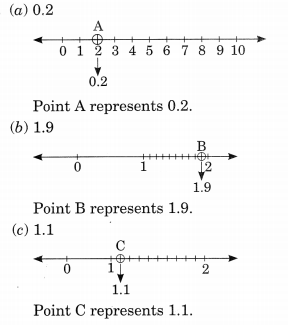Question 9.
Write the decimal number represented by the points A, B, C, D on the given number line.Solution:
Point A represents 0.8
Point B represents 1.3
Point C represents 2.2
Point D represents 2.9

Question 10.
(a) The length of Ramesh’s notebook is 9 cm 5mm. What will be its length in cm?
(6) The length of a young gram plant is 65 mm. Express its length in cm.
Solution:
(a) Length of the notebook = 9 cm 5 mm(b) Length of the young gram plant = 65 mm